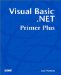# Determining the Size of an Array

 As shown in this chapter, you can increase or decrease the size of a dynamic array according to the needs of the program. Although this offers you great flexibility, there are times when you need to know how many elements are currently defined for the array. After all, if you try to access an element that is larger than the array is dimensioned for, you get a runtime error message from Visual Basic .NET. In such situations, it would be nice to know exactly how large the array is. Being armed with information about the size of an array allows you to write code that can trap values that could cause errors in the program. Such defensive coding is called error trapping. You will learn a lot more about error trapping in Chapter 19, "Error Processing and Debugging." To determine the size of the dynamic array you created earlier, you can add the following new lines to the btnShowDay object's Click() event: ` Dim MyArray( , ) As Integer Dim lo As Integer, hi As Integer ReDim MyArray(5, 3) hi = UBound(MyArray) lo = LBound(MyArray) ` The first line simply defines a two-dimensional dynamic array of integers named MyArray() . The second line defines two new integer variables that you can use in the program. The third line redimensions the dynamic array to have six rows and four columns . (Remember that the numbers in the Dim and ReDim statements are the upper limits of valid index values. And because arrays begin with element 0, you always get one more element than it appears you have defined.) The UBound() function returns the largest valid row index number of the MyArray() array. In our example, the variable hi would be assigned the value 5 . The UBound() value is always equal to the first dimension of the array. That is, if you reversed the dimensions so that the ReDim statement is read as follows : ` ReDim MyArray(3, 5) ` the variable hi would equal 3 , not 5 . This would be true regardless of the number of dimensions in the array. The next program statement uses the LBound() function to return the index value of the lowest valid index number for the DaysOfTheWeek() array. Actually, LBound() is a lot less useful in Visual Basic .NET than it is in earlier releases of Visual Basic because the lower boundary for all arrays in Visual Basic .NET is now always . Remember that earlier releases of Visual Basic allow you to set the lower boundary value for the array to something other than . Armed with information about the lower and upper bounds of the array (as stored in the values for lo and hi ), you can check the value entered by the user before you use it to index into the array. You will see exactly how to write such error trapping code in Chapter 19. For now, you just need to know that there is a way to determine the size of an array while the program is running.Visual Basic .NET Primer Plus
ISBN: 0672324857
EAN: 2147483647
Year: 2003
Pages: 238
Authors: Jack Purdum

Similar book on Amazon